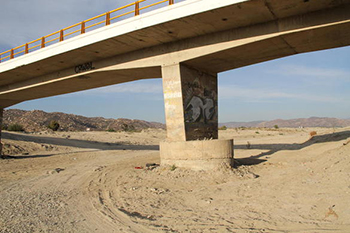online_contraction_scour_live_bed:  Contraction scour under live bed conditions
using modified Laursen 1960 equationModified Laursen formula

ys / y1 = ( Q2 / Q1 ) 0.86 (W1 / [W2) k1  -  ( yo / y1 )

[ yo, y1, Q and W in any consistent set of units ]
[ S1 in m/m or ft/ft ]
[ T in °C or °F ]
[ D50 in mm or ft ]

Reference:

 DESCRIPTION:      [Sample Input]     [Main Page]

To determine contraction scour depth under live bed conditions ys, the calculator requires the following information:

• Mean flow depth below the bridge, before scour yo, in m (or ft).

• Mean flow depth upstream of the bridge y1, in m (or ft).

• Discharge upstream of the bridge (only in the main channel) Q1 in m3/s, or (or cfs).

• Discharge in the contracted section Q2 in m3/s, or (or cfs).

• Bottom width in the section upstream of the bridge W1, in m (or ft).

• Bottom width in the contracted section, excluding piers W2, in m (or ft).

• Energy slope in the main channel upstream S1, in m/m (or ft/ft).

• Median diameter of the bed material D50, in mm (or ft).

• Water temperature θ , in oC (or oF).

1. To determine K1, first calculate the shear velocity in the upstream section V*, and the fall velocity of bed material ω.

1.1. The shear velocity V* is calculated using the formula V* = (gy1S1)1/2

1.2. The fall velocity ω is determined using the graph in Figure 1.Figure 1.  Fall velocity of bed material.

1.3. Finally, with V* and ω, K1 can be defined using Table 1.

 Table 1.  Exponent K1 V* / ω Mode of bed material transport K1 < 0.5 Mostly contact bed material discharge 0.59 0.5 - 2.0 Some suspended bed material discharge 0.64 > 2.0 Mostly suspended bed material discharge 0.69

2. The contraction scour depth ys can then be calculated using the following equation:

 ys           Q2              W1             yo _____  =  (____) 0.86   (____) k1  -  ____   y1           Q1              W2             y1

yo = Given

y1 = Given

Q1 = Given

Q2 = Given

W1 = Given

W2 = Given

k1 = Calculated in step 1.